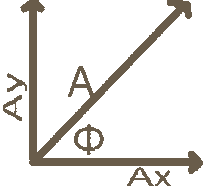# Two Dimensional Vector Magnitude Calculation

Calculate magnitude of 2D vectors (Two Dimensional Vector) for given values:

 Vector A:

 Magnitude:

The magnitude of a vector can be calculated by taking the square root of the sum of the squares of its components. The formula for the magnitude or length of a 2D vector is the Pythagorean Formula.The magnitude of vector is described as the length of physical quantities which have both magnitude and direction. The magnitude or length of any two dimensional vector is denoted by |A|.

The line length shows the magnitude of the vector and arrowhead points towards direction.

The vector can be numerically represented in the Cartesian co-ordinate system as A = (Ax , Ay).

suppose Ax = 5 and Ay = 6

Hence A = (5, 6).

Let’s find the magnitude of vector A

The formula for the magnitude of vector A is |A| = sqrt(x^2 + y^2)

Therefore: |A| = sqrt[5^2 + 6^2]

= sqrt (25 + 36)

= sqrt(61)

= 7.81